/

### Extreme Events – Specimen Question A.5.2(b) – Answer/Hints

Q. Suppose that she is just about to adjust her portfolio mix so that it includes no holding in A3 and with the amounts that were invested in A3 in the above table redistributed equally between the remaining four asset categories. What return assumptions might she be adopting when choosing her new portfolio mix? Can you specify mathematically all possible sets of return assumptions she could have adopted and still reached this answer?

A similar approach as used in Question A.5.2(a) can be used here, but with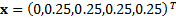rather than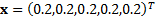and with one further important difference. This is that it is impossible to identify precisely the magnitude of the implied alpha for the first asset class relative to the others – we merely know that it is worse than a certain value, since the no short-selling constraint (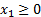applies to it.

To solve this question we therefore need to select a very negative return for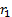and find implied alphas for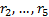as if we only had a 4 asset problem. We would then carry out optimisation exercises using these values for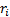but selectively increasinguntil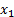just starts to become positive for the chosen value of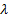. This has introduced a further parameter in the specification of possible asset mixes, but one that is constrained to fall below a given value.

N.B. Conceptually the same sort of approach can be used if we have more than one inequality biting although the trial and error approach used above would soon become relatively impractical.